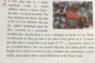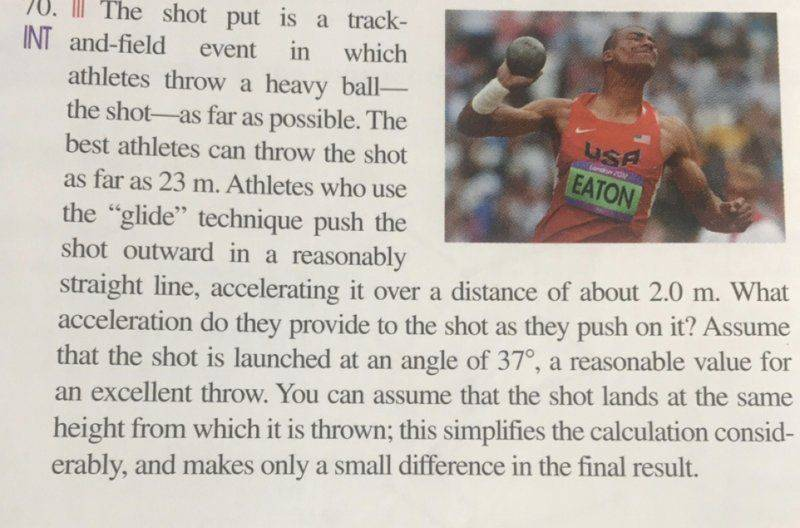# Acceleration of a shot put ball

• Blocky970

## Homework Statement

looking for the acceleration a person exerts on a shot put ball. total distance equals 23m. Thrown at an angle of 37 degrees and accelerating it at a distance of over two meters.

## Homework Equations

I am working with a group of three other girls who are just as lost as i am.

## The Attempt at a Solution

My attempted solution was to find the initial velocity first and use the kinematic equations to solve for Time and other variables which would lead to its acceleration.

#### Attachments

•IMG_4686.jpg
59.6 KB · Views: 359

First, a "shot put ball" is actually a "shot", and the "put" is the style of throw.

How did you use the kinematics equations? We need to see what you've done to see where you are going wrong.

## The Attempt at a Solution

My attempted solution was to find the initial velocity first and use the kinematic equations to solve for Time and other variables which would lead to its acceleration.
Write your equations of movement for X, Y, Vx and Vy, and solve for calculate the velocity just after the shot.
After that think about how the athlete reaches this velocity. What are the equations in this case?

## Homework Statement

looking for the acceleration a person exerts on a shot put ball. total distance equals 23m. Thrown at an angle of 37 degrees and accelerating it at a distance of over two meters.

## Homework Equations

I am working with a group of three other girls who are just as lost as i am.

## The Attempt at a Solution

My attempted solution was to find the initial velocity first and use the kinematic equations to solve for Time and other variables which would lead to its acceleration.
Hello @Blocky970 . Welcome to PF !Have you studied "Projectile Motion" ? kinematic equations ? Newton's Laws ?